## 推导的思路

OpenGL的可视区域的坐标范围是一个边长为2的立方体。每个维度上的大小是2，范围是[-1,+1]。经过各种变换之后的坐标超出[-1,+1]范围的部分将不会显示到屏幕上。

## 正交投影

### 矩阵定义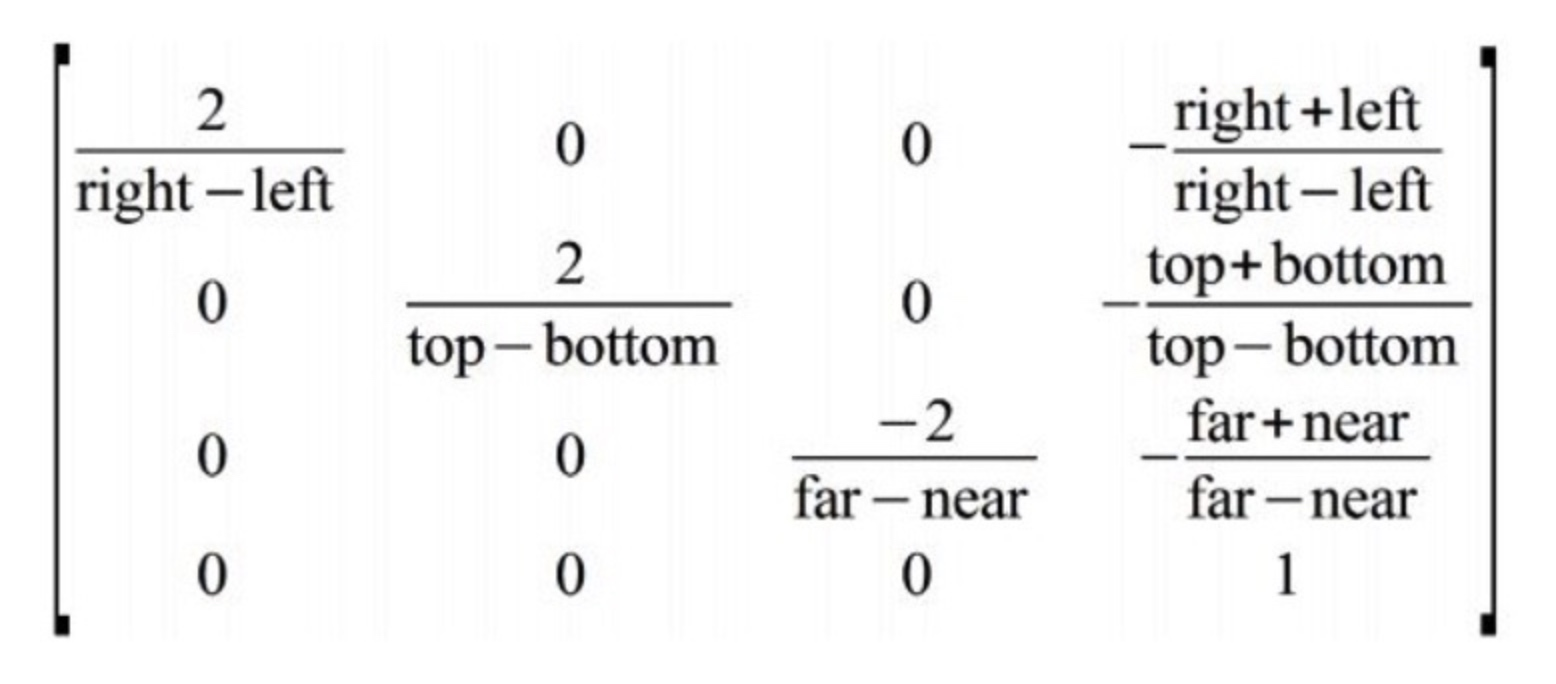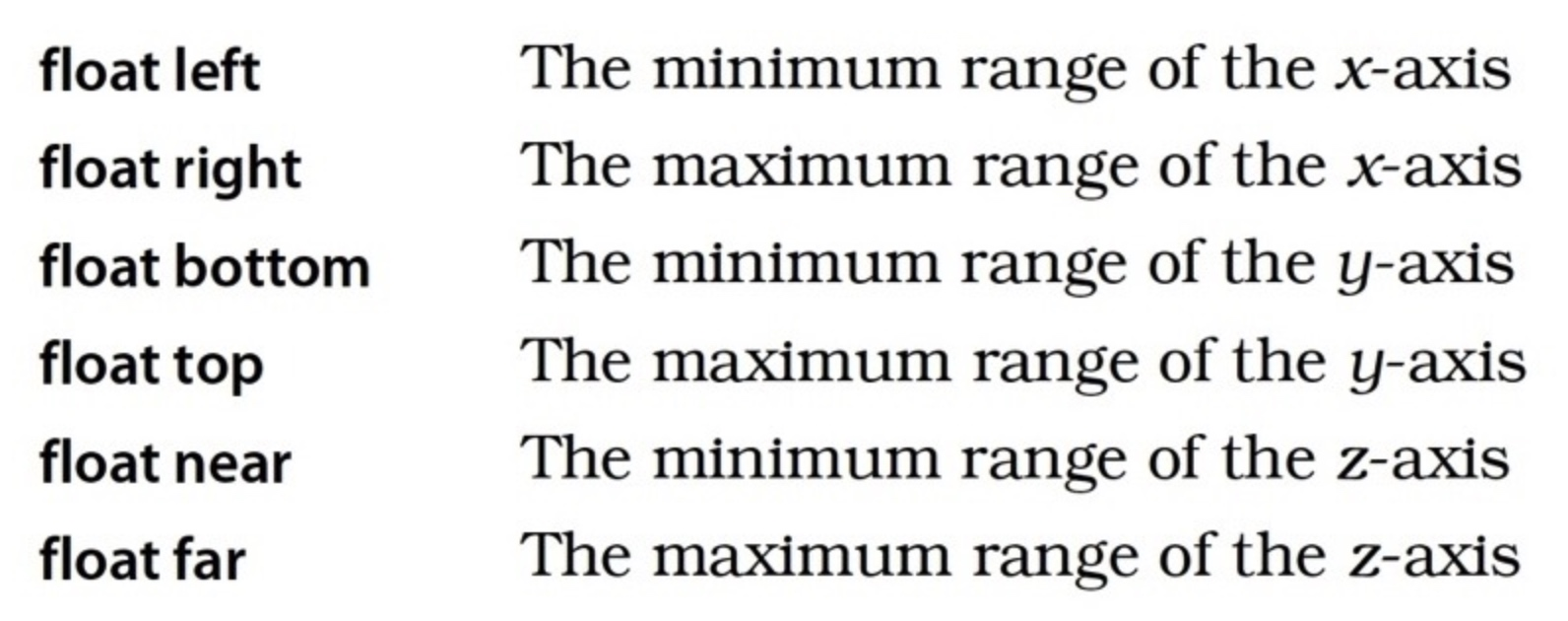$\begin{cases} x \in [left, right]& \text{}\\ y \in [top, bottom]& \text{} \end{cases}$

### 数学推导

① 假设物体上的一个坐标为(x,y,z,1)，其中，x的范围为[left, right]，y的范围为[top, bottom]，z的范围为[near, far]

$\left[ \begin{array}{cccc} \frac{2}{right-left} & 0 & 0 & -\frac{right+left}{right-left}\\ 0 & \frac{2}{top-bottom} & 0 & -\frac{top+bottom}{top-bottom}\\ 0 & 0 & \frac{-2}{far-near} & -\frac{far+near}{far-near}\\ 0 & 0 & 0 & 1 \end{array} \right]× \left[ \begin{array}{c} x\\ y\\ z\\ 1 \end{array} \right]= \left[ \begin{array}{c} \frac{2x-left-right}{right-left}\\ \frac{2y-top-bottom}{bottom-top}\\ \frac{2z-near-far}{far-near}\\ 1 \end{array} \right ]$

$x1=\frac{2x-left-right}{right-left}$
$y1=\frac{2y-top-bottom}{bottom-top}$
$z1=\frac{2z-near-far}{far-near}$
$w1=1$

② 考虑到perspective divide的存在，此时w=1，所以：

$x1=\frac{2x-left-right}{(right-left)w1}=\frac{2x-left-right}{right-left}$
$y1=\frac{2y-top-bottom}{(bottom-top)w1}=\frac{2y-top-bottom}{bottom-top}$
$z1=\frac{2z-near-far}{(far-near)w1}=\frac{2z-near-far}{far-near}$

② 先证明x轴确实落在了[-1, +1]的范围。

$x1=f(x)=\frac{2*x-left-right}{right-left}$

$f(x)=\begin{cases} f(x) = -1,& \text{x=left}\\ f(x) = 1,& \text{x=right} \end{cases}$

③ 所以

$x1=f(x)\in [-1, +1]$

### 小结

$\begin{cases} x \in [left, right],& \text{}\\ y \in [bottom, top],& \text{}\\ z \in [near, far],& \text{} \end{cases}$

$\begin{cases} x \in [-1, 1],& \text{}\\ y \in [-1, 1],& \text{}\\ z \in [-1, 1],& \text{} \end{cases}$

## 透视投影

### 变换效果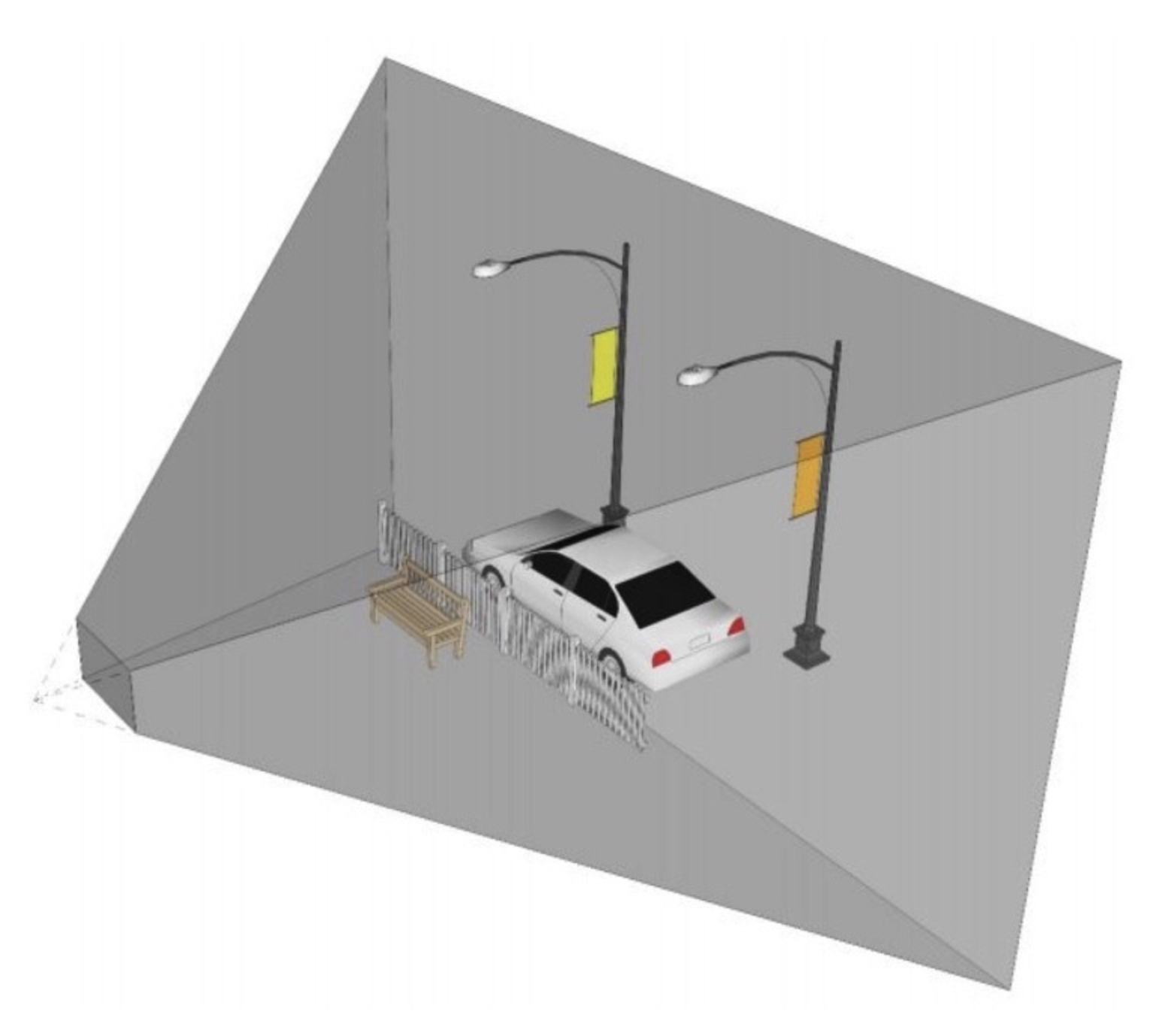### 矩阵定义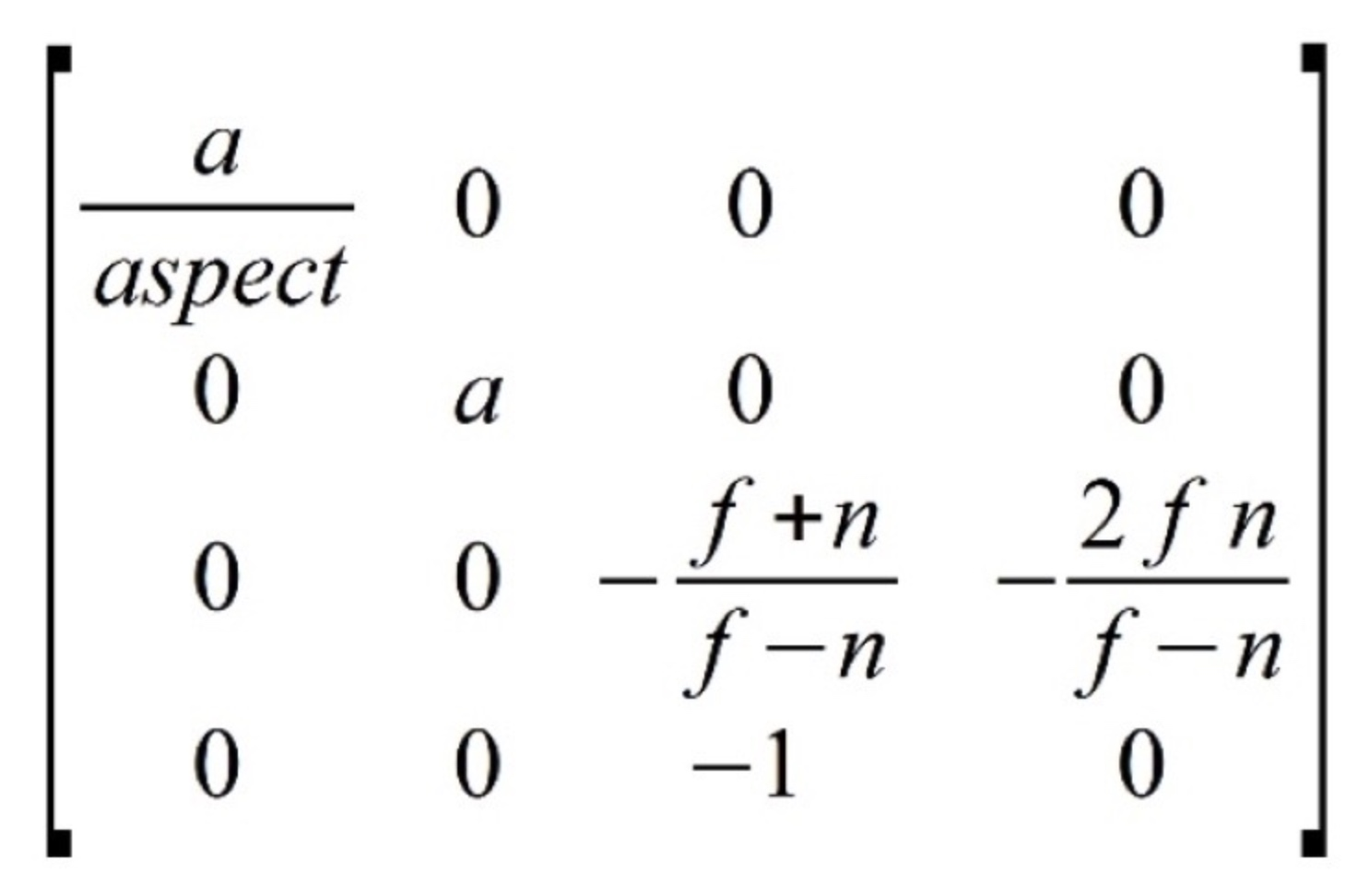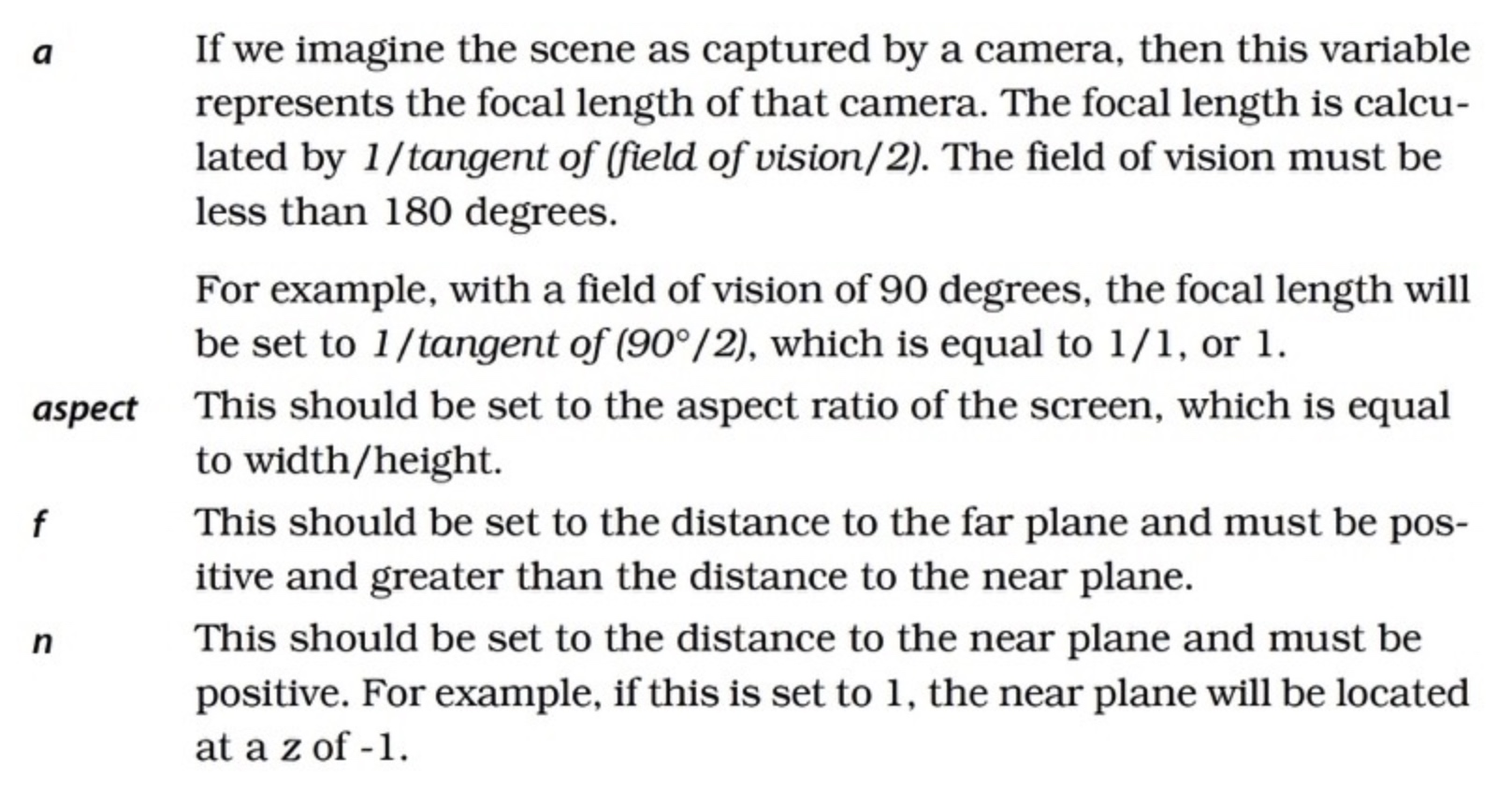### 数学推导

① 假设物体上的一个坐标为(x,y,z,1)

$\left[ \begin{array}{cccc} \frac{a}{aspect} & 0 & 0 & 0\\ 0 & a & 0 & 0\\ 0 & 0 & -\frac{f+n}{f-n} & -\frac{2fn}{f-n}\\ 0 & 0 & -1 & 0 \end{array} \right]× \left[ \begin{array}{c} x\\ y\\ z\\ 1 \end{array} \right]= \left[ \begin{array}{c} \frac{ax}{aspect}\\ ay\\ -\frac{f+n+2fnw}{f-n}\\ -z \end{array} \right ]$

$x1=\frac{ax}{aspect}$
$y1=ay$
$z1=-\frac{(f+n)z+2fnw}{f-n}$
$w1=-z$

② 考虑perspective divide的存在，得到:

$x2=\frac{x1}{w1}=\frac{-ax}{aspect * z}$
$y2=\frac{y1}{w1}=-\frac{ay}{z}$
$z2=\frac{z1}{w1}=\frac{(f+n)z+2fn}{(f-n)z}$

③ 求：当结果落在了[-1, +1]的范围的时候，x的范围是多少？

$x2=f(x)=\frac{-ax}{aspect*z}$

$x=\begin{cases} \frac{aspect*z}{a},& \text{f(x)=-1}\\ -\frac{aspect*z}{a},& \text{f(x)=1} \end{cases}$

$[\frac{aspect*z}{a},-\frac{aspect*z}{a}]$

④ 求：当结果落在了[-1, +1]的范围的时候，y的范围是多少？

$y2=f(y)=-\frac{ay}{z}$

$y=\begin{cases} \frac{z}{a},& \text{f(y)=-1}\\ -\frac{z}{a},& \text{f(y)=1} \end{cases}$

$[\frac{z}{a},-\frac{z}{a}]$

⑤ 求：当结果落在了[-1, +1]的范围的时候，z的范围是多少？

$z2=f(z)=\frac{z1}{w1}=\frac{(f+n)z+2fn}{(f-n)z}$

$-1<=f(z)<=1$

$-1<=\frac{(f+n)z+2fn}{(f-n)z}<=1$

$-1<=f(z)<=1$

$n<=z<=f$

### 小结

$\begin{cases} x \in [\frac{aspect*z}{a},-\frac{aspect*z}{a}],& \text{}\\ y \in [\frac{z}{a},-\frac{z}{a}],& \text{}\\ z \in [n,f],& \text{} \end{cases}$

$\begin{cases} x \in [-1, 1],& \text{}\\ y \in [-1, 1],& \text{}\\ z \in [-1, 1],& \text{} \end{cases}$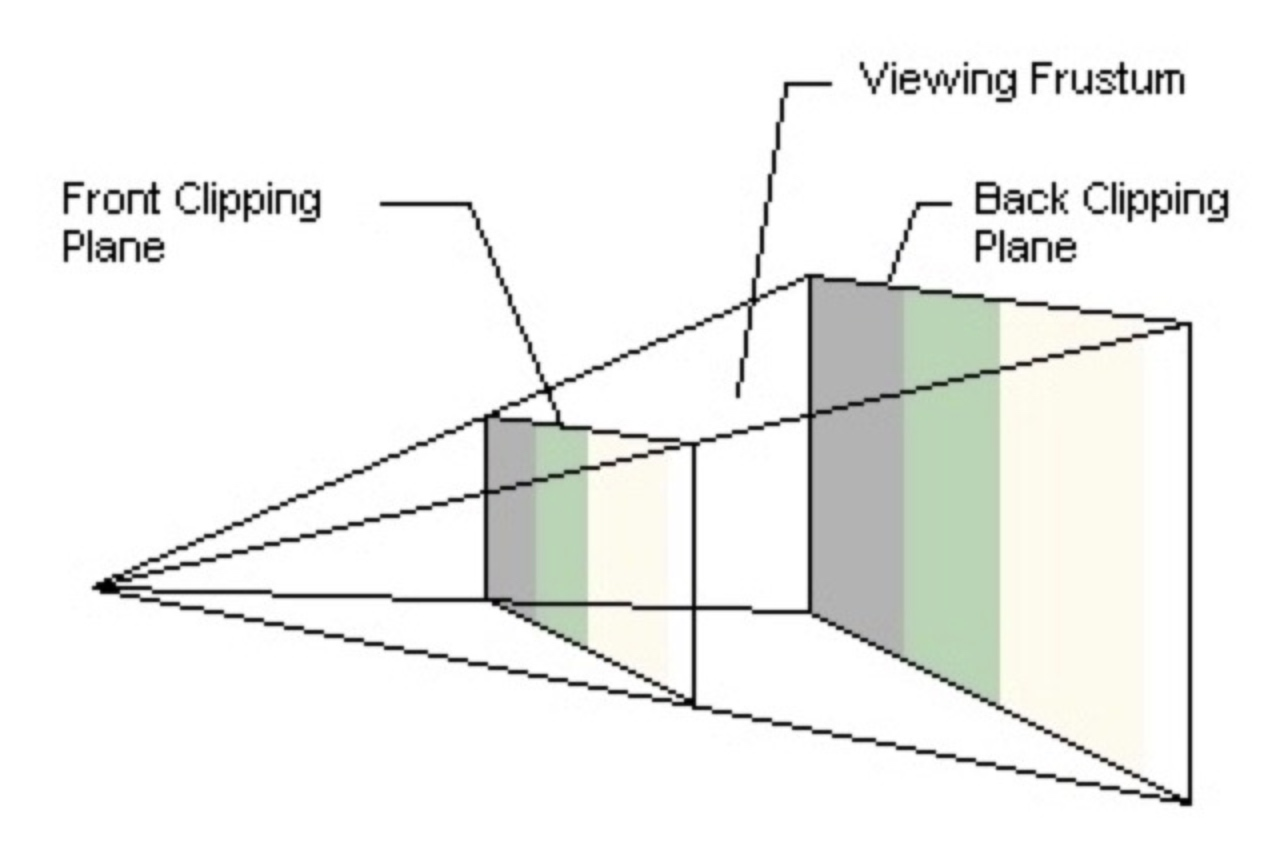## 总结

### 正交变换

$\begin{cases} x \in [left, right],& \text{}\\ y \in [bottom, top],& \text{}\\ z \in [near, far],& \text{} \end{cases}$

$\begin{cases} x \in [-1, 1],& \text{}\\ y \in [-1, 1],& \text{}\\ z \in [-1, 1],& \text{} \end{cases}$

### 透视变换

$\begin{cases} x \in [\frac{aspect*z}{a},-\frac{aspect*z}{a}],& \text{}\\ y \in [\frac{z}{a},-\frac{z}{a}],& \text{}\\ z \in [n,f],& \text{} \end{cases}$

$\begin{cases} x \in [-1, 1],& \text{}\\ y \in [-1, 1],& \text{}\\ z \in [-1, 1],& \text{} \end{cases}$